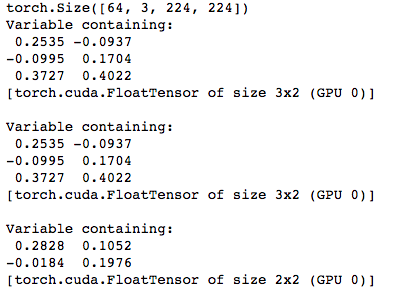# [Solved] Inconsistent results during test using different batch size

I encountered a weird problem when using a one-hidden-layer fully connected network without using batch normalization: the test set performance varies hugely when using different batch sizes. To be clear, I did switch the mode of the network to `mlp.eval()` before doing the actual testing – although I think this does not matter in my case since the network does not have batch normalization, but it does have `dropout`.

Here is the code snippet:

``````    mlp = MLPNet(configs)
if args.cuda:
mlp = mlp.cuda()
mlp.train()
for t in xrange(num_epochs):
running_loss = 0.0
xs, ys = torch.from_numpy(xs), torch.from_numpy(ys)
if args.cuda:
xs, ys = xs.cuda(), ys.cuda()
ypreds = mlp(xs)
loss = F.nll_loss(ypreds, ys)
running_loss += loss.data
loss.backward()
optimizer.step()
logger.info("Iteration {}, loss value = {}".format(t, running_loss))
time_end = time.time()
logger.info("Time used for training on {} = {} seconds.".format(data_name[i], time_end - time_start))
# Test on other data sets.
mlp.eval()
for j in xrange(num_data_sets):
target_idx = j
target_insts = data_insts[j][num_trains:, :].todense().astype(np.float32)
target_labels = data_labels[j][num_trains:, :].ravel().astype(np.int64)
num_corrects = 0.0
xs, ys = torch.from_numpy(xs), torch.from_numpy(ys)
if args.cuda:
xs, ys = xs.cuda(), ys.cuda()
ypreds = mlp(xs)
num_corrects += torch.sum(torch.max(ypreds, 1) == ys).cpu().data
acc = num_corrects / float(target_insts.shape)
``````

what I found is that when I change the `batch_size` in test_loader, the final `acc` will vary drastically. Any ideas on what’s the problem for this?

Hello,

I have the same problem for testing. Could you show how it was solved?

My problem was due to the inconsistent broadcasting of 1d tensors. Just to make sure that your 1d tensor has shape (n,) not (n, 1).

1 Like

Thank you. That solved my problem!

I encountered a similar problem. Different batch sizes gave me different results. model.eval() is used in my testing.

Could you be more specific, which 1d tensor you used is causing the problem?

thanks,

This problem also happens to me, could I ask do you solve it? if yes, how? thanks.

Were you guys able to resolve this? This has been happening to me and it’s really weird. Different batch size is giving different scores for the same input!

print(x_var.size())

``````    scores = model(x_var[0:3])
print(scores)

scores = model(x_var[0:3])
print(scores)

scores = model(x_var[0:2])
print(scores)
``````EDIT: Never mind - figured it out. Make sure to do model.train(False) so Dropout and Batchnorm are in test mode.

3 Likes

Thank you for your solution, it worked for me!